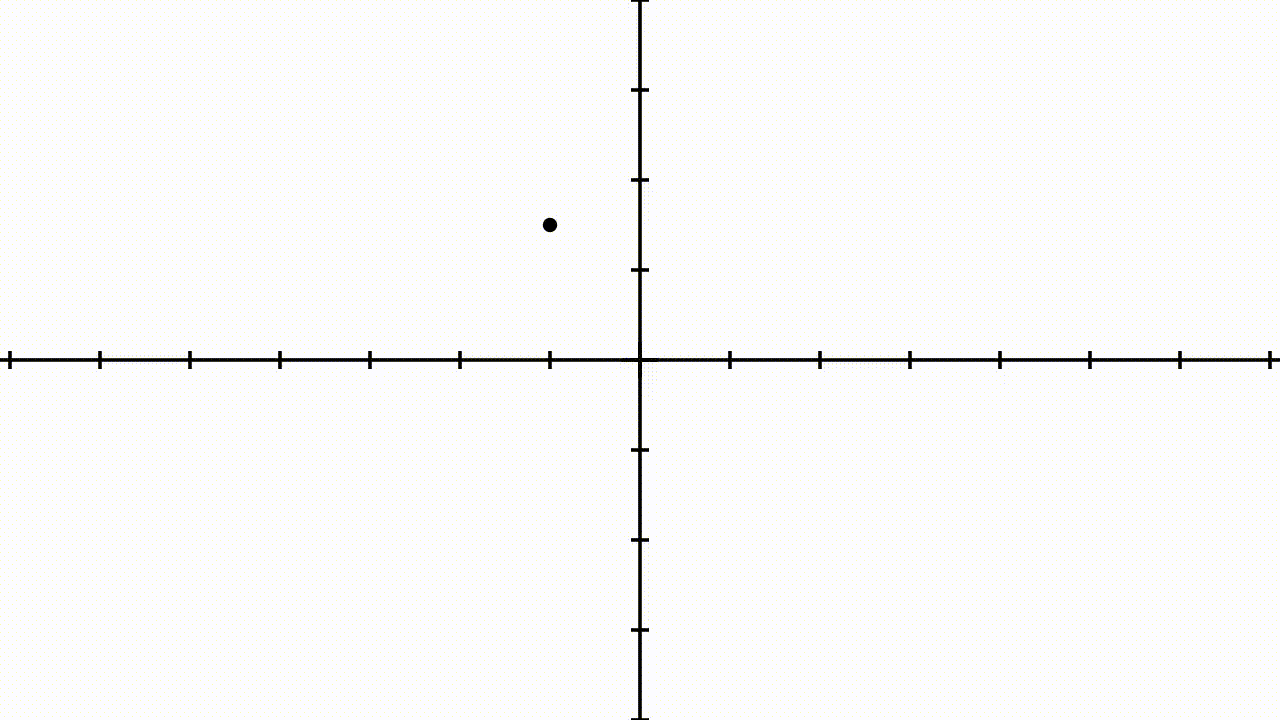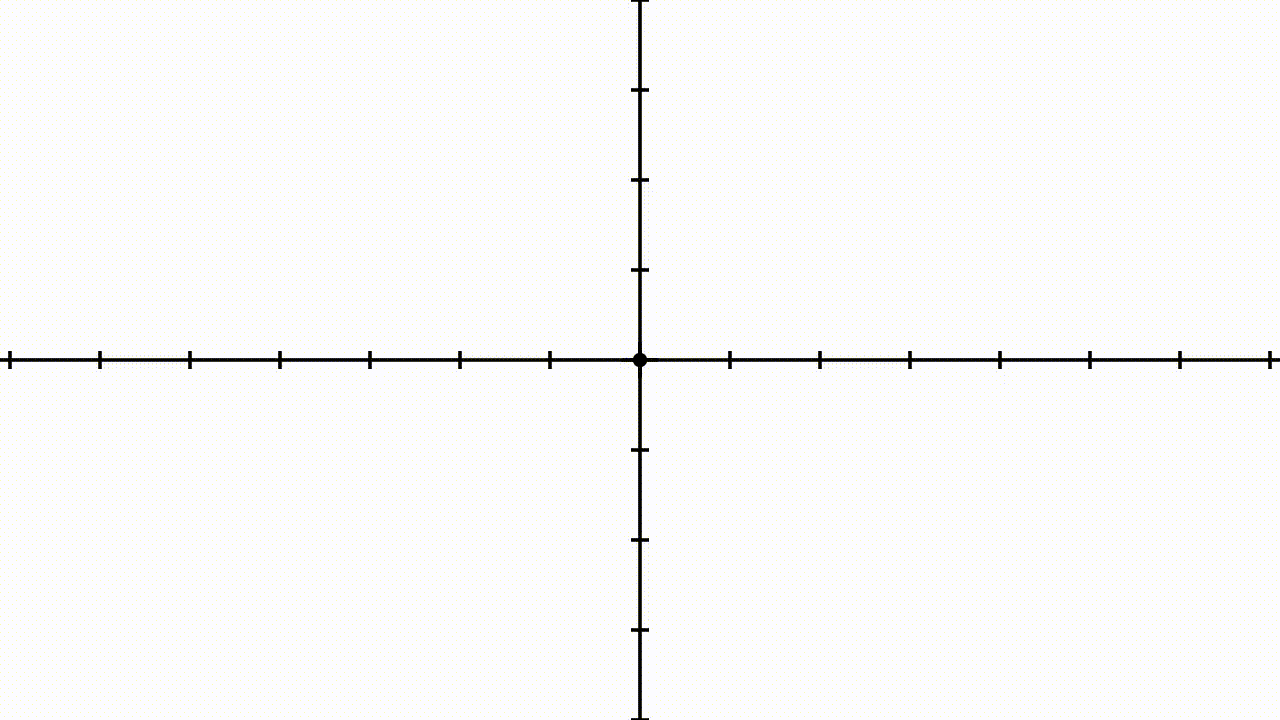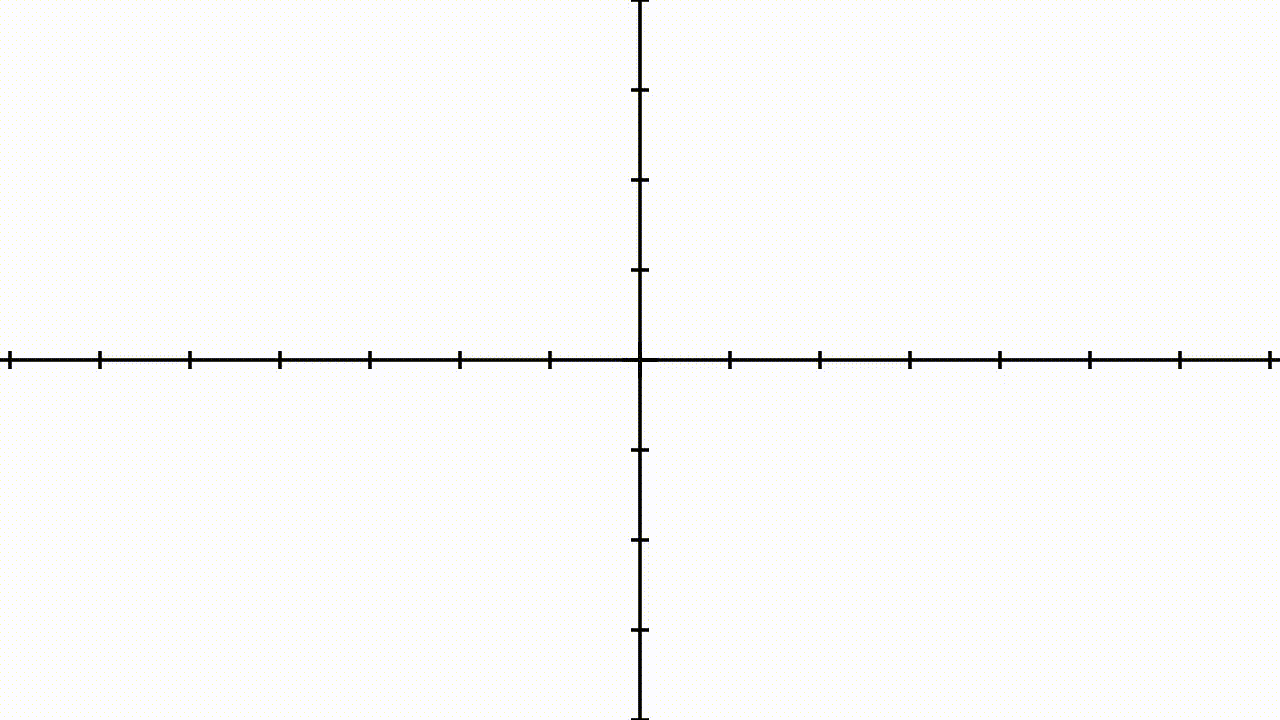## 1. Introduction

In this tutorial, we’ll describe an algorithm for inflating or deflating polygons.

## 2. Homothety in the General Form

Let’s begin with a shape, for simplicity, a square. We’re probably familiar with the idea of scaling a shape by making it larger or smaller. This idea, technically, takes the name of homothetic transformation or homogeneous dilation of the space:We start with any polygon defined by a set ofvertices. Then, we identify a pointthat we call the centre of the homothety. After selecting a homothetic ratio, any real number, we can then compute the corresponding new verticesin the transformed polygon as:If we know the coordinates of the vertices, their corresponding homothetically transformed imagescan be calculated as:## 3. Uniform Scaling as a Special Case

In the special case in which the coordinates of the homothetic center are, the homothety takes place in relation to the origin. This simplifies the calculation of the image of the transformed polygon significantly. Thus, the previous formula is reduced to:This special type of homothetic transformation takes the name of uniform scaling. The factor, in this case, assumes the name of the scaling factor of the transformation.

## 4. Homothetically Transforming Versus Offsetting Shapes

A related problem is the one entailing the offsetting of a shape or polygon. In some cases, we may want to hypothetically transform a polygon but also impose additional constraints on the resulting shape. For example, we may wish to satisfy the added constraint that all points in the perimeter of the new shape are equidistant with their closest points in the perimeter of the original shape:If the original shape is a circle and we scale it around its center, for example, the homothetic transformation respects this rule.

Notice that, however, the homothety does not generally satisfy this constraint. This is because each of the corner points or vertices in a transformed polygon is, in fact, generally farther from its inverse image than any other point in the transformed shape.

The transformation that satisfies the restricted condition takes the name of offsetting of a polygon, also known in the geospatial analysis as polygon buffering.

## 5. Procedure for Offsetting Polygons

To offset a polygon with known vertices, we need an algorithm that performs the following tasks, in order. First, we take each vertex and duplicate it. Then, we connect each new vertex with the old vertex that precedes it in clockwise order.

This lets us define segments that can move independently from one another. After selecting an arbitrary length, we then shift the segments along their axis by that.

By remembering the definition of perpendicularity between segments, we can first compute the slopeof each segment, as:The lines perpendicular to this segment all have a slope that is the reciprocal of the inverse of. For each of the vertices of a segment with slope, the new coordinatesthen need to satisfy these two equations:For. These are two equations in two unknowns, which we can solve to find the new coordinates for each vertex.

## 6. Connecting the Dots

The last remaining step consists of connecting the translated segments with one another. This can be done by creating an arc of the circumference with radius. Each arc is centered on each original vertex and bound within the two images of the duplicated vertices.

This animation shows the full procedure of inflation, from the definition of the polygon to its final shape:Notice that this procedure also works for concave polygons: we need to pay attention, though, to check that all offset vertices lie outside of the transformed polygons. If they don’t, we need to remove them and all the lines that fall within the polygon. We can refer to our tutorial on geofencing if we need a refresher on how to do that.

The procedure also works, mutatis mutandis, to deflate polygons. In this case, we need to invert the direction in which we translate the segments. This can be done by swapping the sign of thefactor that we defined earlier. Then, once again, we delete all points that fall outside of the shape of the newly offset polygon.

## 7. Conclusions

In this article, we studied how to inflate or deflate a polygon utilizing homothety and offsetting.